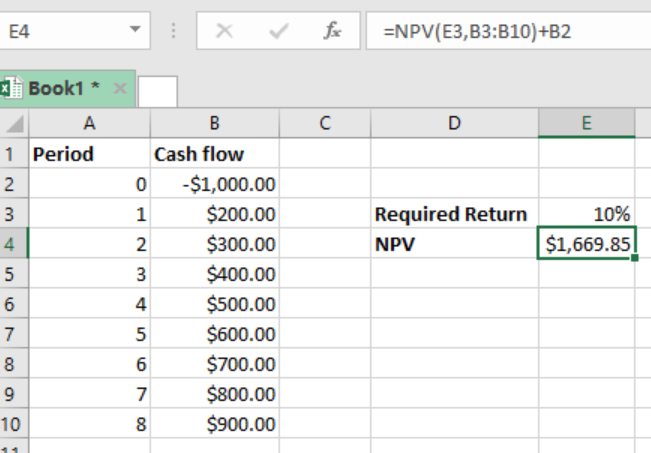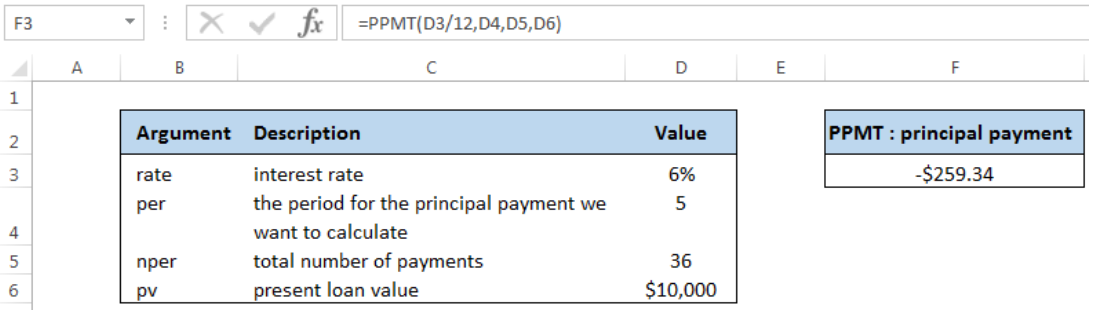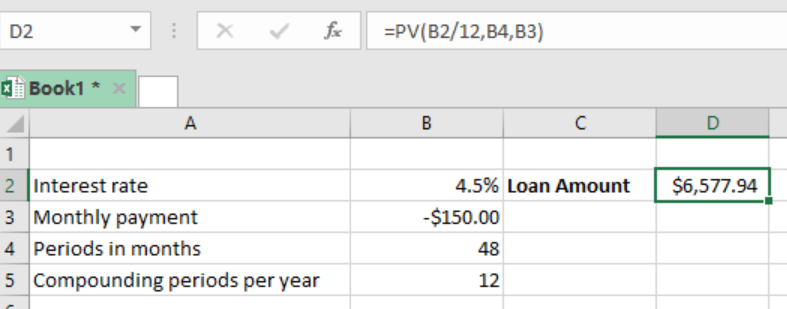Get instant live expert help with Excel or Google Sheets“My Excelchat expert helped me in less than 20 minutes, saving me what would have been 5 hours of work!”

#### Post your problem and you’ll get expert help in seconds.

Your message must be at least 40 characters
Our professional experts are available now. Your privacy is guaranteed.

# Excel Financial Function Examples – Financial Formulas in Excel, Financial Analysis Excel

Whether you’re a business owner, financial consultant, investment advisor, or student, you may have use of the many financial analysis functions and formulas available in Excel. In fact, this is one of the strengths of this software.

Excel provides its users with a variety of ways to perform financial calculations, from the simple to the complex. Here is a list of the financial tutorials available on Excelchat. If you need something more or don’t want to search through this list, our live chat feature is available for problem-specific help.Learn about Excel’s financial functions from these tutorials.

## How to Calculate Simple Interest in Excel

This tutorial explains how you can use Excel to calculate simple interest when the principal, rate, and term are provided. It provides the formula to accomplish this, an explanation of the formula, and three different examples with illustrations.

## The Interest Rate Function in Excel

This piece shows the reader how to use the PMT function in Excel to calculate an interest rate. It provides the syntax of a formula using PMT and then includes some example. There is also a detailed video tutorial for additional guidance.

## How to Calculate Cumulative Loan Principal Payments in Excel

This tutorial gives the reader information about using the CUMPRINC function in Excel to calculate principal loan payments. The reader receives the formula using CUMPRINC as well as an explanation of the parameters and an example of its use.

## How to Calculate an Original Loan Amount in Excel

This piece explains how you can use Excel to calculate the original amount of a loan by using the PV function. It illustrates how to set up your data, use PV in a formula, and provides an example with illustrations as a guide.

## Excel NPV Function

This is a guide on using the NPV function in Excel to determine the net present value of a cash flow series with a given discount rate. The reader will receive the formula using NPV, an explanation of terms, and a detailed example with illustrations.## How to Use the Excel PPMT Function

This is a tutorial on using the PPMT function in Excel to determine the principal payment for a loan given the other set of terms. The piece gives the syntax for the formula using PPMT, shows how to set up the data, and provides a detailed example.

## Annual Compound Interest Schedule in Excel

This piece explains how the reader can create an annual compound interest schedule in Excel using a formula. Instead of a function, this tutorial shows how to set up your data and then apply a particular formula to get the desired result. An example is included.

## Excel NPER Function

This guide shows the reader how to find the number of installments paid within a given period by using the NPER function. This function requires that other data be available, such as the rate, payment, and present value. An example is provided.

## Excel XIRR Function

This is a tutorial on using the XIRR function in Excel to calculate the internal rate of return for scheduled cash flows that may not be periodic cash flows. It provides the syntax for the formula using XIRR, an explanation of the terms, and a detailed example with illustrations.

## Calculate Principal for Given Period

This piece will show the reader how to find the principal payment for a loan using the PPMT function in Excel. It explains what data is required for this to work, gives the syntax for the formula, and provides an example with illustrations.## Excel RATE Function

This tutorial explains how to use Excel’s RATE function to work with interest rates. Specifically, this financial function can be used to determine the interest rate on a loan when other terms are provided. The syntax and an example with illustrations are provided.

## Calculate Cumulative Loan Interest

This is a guide on calculating the cumulative interest paid on a loan using the CUMIPMT function in Excel. After setting up the data, the generic formula using CUMIPMT is provided, and then there is a detailed example with illustrations.

## Excel CUMPRINC Function

This piece explains how the reader can determine how much of their loan’s principal they have paid off by using the CUMPRINC function in Excel. It illustrates how to properly set up the data, provides the formula syntax, and then gives an example.

## Calculate Interest for Given Period

This tutorial explains how you can calculate the interest for a loan during a given period using the IMPT function. It provides the generic formula for this function as well as a detailed example with illustrations.

## Excel PV Function

This is a high-level piece on using the PV function in Excel to determine the present value of an investment. Once provided with the required data for the calculation, this tutorial shows how to use the PV formula in an example.## Excel FV Function

This tutorial shows the reader how to use the FV function in Excel to find the future value of an investment. It explains what data is required, gives the formula syntax, and provides a detailed example.

## Excel IRR Function

This is a piece that outlines the use of the IRR function in Excel to determine the internal rate of return of an investment. It shows the reader how to set up the data, provides the generic formula using IRR, and gives a detailed example.

## How to Calculate Payment For a Loan in Excel

In this guide, you’ll learn how to calculate the payment for a loan in Excel using the PMT function. The tutorial explains what other data is required and gives the generic formula using PMT. It then shows how this works with a detailed example.

Were you able to find the Excel tutorial you needed to learn about financial functions or troubleshoot your issue? Whether this list fell short or you didn’t want to take the time to sort through several guides, there are other assistance options available.

Excelchat can connect with you immediately through live chat to teach you about Excel or walk you through solving a troubling issue. Just click on the live chat feature and one of our knowledgeable and friendly Excel experts will be there to provide one-on-one assistance. Your first session is always free.